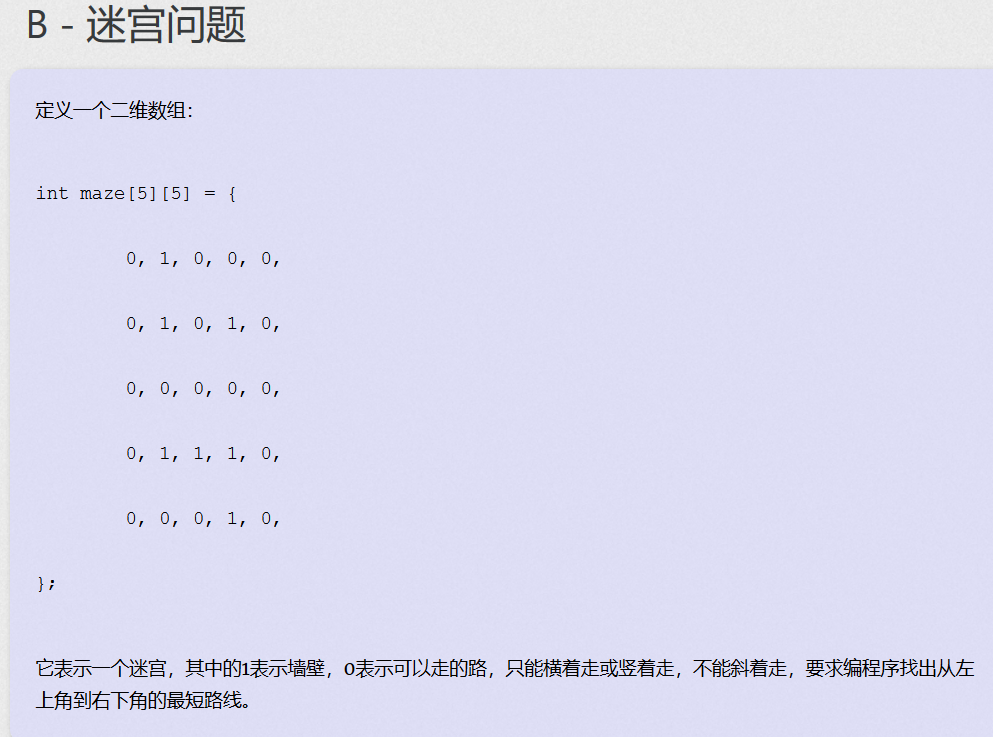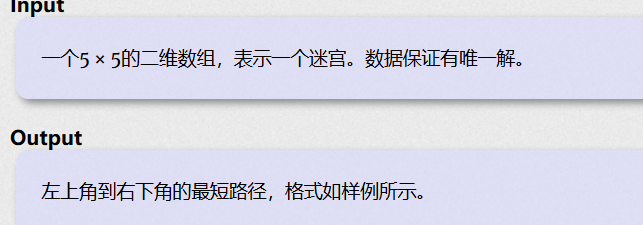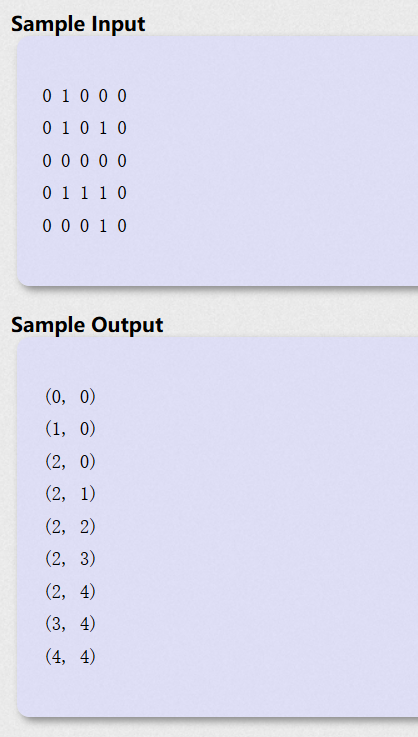## DFS

DFS即深度搜索，每次都沿着路径到不能再前进的时候才退回到最近的岔口。具体不再详细论述。

（1）连通分量： 在无向图中，如果有两个顶点可以相互到达（可以是通过一定的路径间接到达）那么就称这两个顶点连通。如果图中任意两个点都连通，则称图G为连通图；否则称它为非连通图，且称其中的极大连通子图为连通分量。
（2）强连通分量：在有向图中，如果两个顶点可以各自通过一条有向路径到达另一个顶点，就称这两个顶点强连通。如果图中任意两个顶点都强连通，则称图为强连通图；否则称图为非强连通图，且称其中的极大强连通子图为强连通分量。

``````DFS(u){

vis[u]=true;
for(从u出发的所有顶点v) //枚举从u出发可以到达的所有顶点v
if vis[v]=false
DFS(v);
}
DFSTrave(G){

for(G的所有顶点u）//对G的所有顶点u
if vis[u]==false
DFS(u);
}
``````

``````int n,G[maxn][maxn];
bool vis[maxn]={
false};

void DFS(int u,int depth)
{

vis[u]=true;
for(int v=0;v<n;v++)
{

if(vis[v]==false&&G[u][v]!=inf)
DFS(v,depth+1);
}
}

void DFSTrave()
{

for(int u=0;u<n;u++)
if(vis[u]==false)
DFS(u,1);
}
``````

``````vector<int> Adj[maxn];
int n;
bool vis[maxn]={
false};

void DFS(int u,int depth)
{

vis[u]=false;
{

if(vis[v]==false)
DFS(v,depth+1);
}
}

void DFSTrave()
{

for(int u=0;u<n;u++)
if(vis[u]==false)
DFS(u,1);
}
``````

## BFS

``````BFS(u)
{

queue q;
将u入队；
inq[u]=true;
while(q非空)
{

取出q的队首元素u进行访问；
for(从u出发可达的所有顶点v)
{

if(inf[v]==false)
{

将v入队;
inq[v]=true;
}
}
}
}
``````

``````int n,G[maxn][maxn];
bool inq[maxn]={
false};

void BFS(int u)
{

queue <int> q;
q.push(u);
inq[u]=true;
while(!q.empty())
{

int u=q.front();
q.pop();
for(int v=0;v<n;v++)
{

if(inq[v]==false&&G[u][v]!INF)
{

q.push(v);
inq[v]=true;
}
}
}
}
void BFSTrave()
{

for(int u=0;u<n;u++)
{

if(inq[u]==false)
BFS(q);
}
}
``````

``````vector<int> Adj[maxn];
int n;
bool inq[amxn]={
false};

void BFS(int u)
{

queue<int> q;
q.push(u);
inq[u]=true;
while(!q.empty())
{

int u=q.front();
q.pop();
{

if(inq[v]==false)
{

q.push(v);
inq[v]=true;
}
}
}
}
void BFSTrave()
{

for(int u=0;u<n;u++)
{

if(inq[u]==false)
{

BFS(q);
}
}
}
````````````#include<iostream>
#include<queue>
using namespace std;
int m;
int dy={
0,0,1,-1};
int dx={
1,-1,0,0};
struct f
{

int x,y;
int num;
int path;
}en;
int k;
void bfs( )
{

queue<f> q;
while(!q.empty())
{

temp=q.front();
q.pop();
for(int i=0;i<4;i++)
{

now.x=temp.x+dx[i];
now.y=temp.y+dy[i];
if(now.x<0||now.x>4||now.y<0||now.y>4||m[now.x][now.y]==1)
continue;
if(m[now.x][now.y]==0)
{

m[now.x][now.y]=1;
now.path[temp.num ]=temp.x;
now.path[temp.num ]=temp.y;
now.num=temp.num +1;
q.push(now);
if(now.x==4&&now.y==4)
{

now.path[now.num]=now.x;
now.path[now.num]=now.y;
now.num=now.num+1;
en=now;
return ;
}
}
}
}
}
int main( )
{

for(int i=0;i<5;i++)
for(int j=0;j<5;j++)
cin>>m[i][j];
bfs();
for(int i=0;i<en.num;i++)
cout<<"("<<en.path[i]<<", "<<en.path[i]<<")"<<endl;
return 0;
}
``````

### 使用IcoMoon将svg图片生成字体图标_敲代码有瘾的博客-程序员宝宝

1.进入IcoMoon官网 https://icomoon.io/点击iconmoon App按钮2.点击import icons按钮3.选中你的svg图片，然后点击Generate Font4.这里可以配置一些图标信息，然后点击下载5.下载完成解压后只需要将fonts和style.css保留就ok6.使用...

### Android3.0升级后出现ButterKnife失效报错的问题解决_ok406lhq的博客-程序员宝宝

Error:Execution failed for task ':reading_routine:javaPreCompileDebug'.&amp;gt; Annotation processors must be explicitly declared now. The following dependencies on the compile classpath are found to co...

### Activiti工作流之流程变量_空城1995的博客-程序员宝宝

1.什么是流程变量流程变量在 activiti 中是一个非常重要的角色，流程运转有时需要靠流程变量，业务系统和 activiti 结合时少不了流程变量，流程变量就是 activiti 在管理工作流时根据管理需要而设置的变量。比如在请假流程流转时如果请假天数大于 3 天则由总经理审核，否则由人事直接审核，请假天数就可以设置为流程变量，在流程流转时使用。注意：如果将 pojo 存储到流...